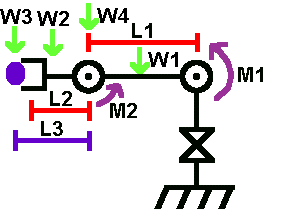###Author Topic: Leg torque question  (Read 7017 times)

0 Members and 1 Guest are viewing this topic.

####silent tone• Beginner
•• Posts: 4##### Leg torque question
« on: March 05, 2007, 10:04:23 PM »
I've looked through the static mechanics guide and still have a question before I start making purchases.
My design includes 2 jointed legs with a servo at the hip and knee.  When the upper leg is parallel to the ground, I'll need the most torque.  If I assume that the foot is secure without slipping and the other leg can oppose any lateral forces (keeping the torso from translating side to side), then can the knee servo provide any vertical force to assist the hip servo in holding the robots weight up?  To maybe be clearer, the knee is applying a torque on the upper leg to rotate it to the vertical above the knee and the hip is appling a torque to rotate the thigh to the vertical, beneath the hip.

I know this isn't applicable for an arm where the only anchor point is the base and the actuator there must handle all the torque for an extended arm.  But on a leg, the foot isn't dangling out in the air.

So, if I'm right, the formula is:

max body weight (supportable by one leg) = (sin(Angle[knee]) * Torque[knee] + sin(Angle[hip]) * Torque[hip]) / upper leg length

And if,
upper leg length = 1 ft
hip torque = 2 ft-lb
knee torque = 1 ft-lb
knee angle is 90
hip angle is 90
(entire leg + actuators have 0 mass)

then,
max body weight is 3 lbs right?

Thanks

####Admin##### Re: Leg torque question
« Reply #1 on: March 07, 2007, 02:28:15 PM »
You can actually look at it as a robot arm. The foot would be the base, the knee the base joint, and the hip another joint on the arm.

As such, the knee would be experiencing the highest torque.

So to calculate, its just like this image (M1 is knee torque and M2 is hip torque):so the equation at 90 degrees for the knee joint is (assuming L2 is 0):
force * length = knee torque
weight = (1 ft-lb)/(1 ft) = 1 lb

this is only static torque, however. this does not account for dynamic torque (moving a mass). what you want to do is assume the knee joint is the center of a wheel, and the upper leg length is the radius of a wheel. then use my dynamics tutorial to select the appropriate motor. this way you can also choose your leg velocity and acceleration.

hopefully i didnt over complicate it for you!